6265.0 - Underemployed Workers, Australia, Sep 2007
ARCHIVED ISSUE Released at 11:30 AM (CANBERRA TIME) 25/02/2008
Page tools:Print All

TECHNICAL NOTE DATA QUALITY

INTRODUCTION

1 Since the estimates in this publication are based on information obtained from occupants of a sample of dwellings, they are subject to sampling variability. That is, they may differ from those estimates that would have been produced if all dwellings had been included in the survey. One measure of the likely difference is given by the standard error (SE), which indicates the extent to which an estimate might have varied by chance because only a sample of dwellings was included. There are about two chances in three (67%) that a sample estimate will differ by less than one SE from the number that would have been obtained if all dwellings had been included, and about 19 chances in 20 (95%) that the difference will be less than two SEs. Another measure of the likely difference is the relative standard error (RSE), which is obtained by expressing the SE as a percentage of the estimate.

2 Due to space limitations, it is impractical to print the SE of each estimate in the publication. Instead, a table of SEs is provided to enable readers to determine the SE for an estimate from the size of that estimate (see table T1). The SE table is derived from a mathematical model, referred to as the 'SE model', which is created using data from a number of past Labour Force Surveys. It should be noted that the SE model only gives an approximate value for the SE for any particular estimate, since there is some minor variation between SEs for different estimates of the same size.

CALCULATION OF STANDARD ERROR

3 An example of the calculation and the use of SEs in relation to estimates of persons is as follows. Table 5 shows the estimated number of female underemployed part-time workers was 300,300. Since this estimate is between 300,000 and 500,000, table T1 shows that the SE for Australia will lie between 5,950 and 7,500 and can be approximated by interpolation using the following general formula: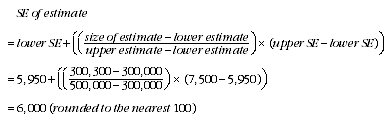4 Therefore, there are about two chances in three that the value that would have been produced if all dwellings had been included in the survey will fall within the range 294,300 to 306,300 and about 19 chances in 20 that the value will fall within the range 288,300 to 312,300. This example is illustrated in the following diagram.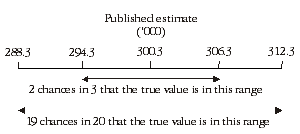5 In general, the size of the SE increases as the size of the estimate increases. Conversely, the RSE decreases as the size of the estimate increases. Very small estimates are thus subject to such high RSEs that their value for most practical purposes is unreliable. In the tables in this publication, only estimates with RSEs of 25% or less are considered reliable for most purposes. Estimates with RSEs greater than 25% but less than or equal to 50% are preceded by an asterisk (e.g.*3.2) to indicate they are subject to high SEs and should be used with caution. Estimates with RSEs of greater than 50%, preceded by a double asterisk (e.g.**0.3), are considered too unreliable for general use and should only be used to aggregate with other estimates to provide derived estimates with RSEs of less than 25%.

MEANS AND MEDIANS

6 The RSEs of estimates of mean duration of insufficient work, median duration of insufficient work and mean preferred number of extra hours are obtained by first finding the RSE of the estimate of the total number of persons contributing to the mean or median (see table T1) and then multiplying the resulting number by the following factors:

• mean duration of insufficient work: 1.6
• median duration of insufficient work: 2.5
• mean preferred number of extra hours: 0.7

7 The following is an example of the calculation of SEs where the use of a factor is required. Table 5 shows that the estimated number of male underemployed part-time workers was 171,800 with a median duration of insufficient work of 25 weeks. The SE of 171,800 can be calculated from table T1 (by interpolation) as 4,700. To convert this to an RSE we express the SE as a percentage of the estimate or 4,700/171,800 =2.7%.

8 The RSE of the estimate of median duration of insufficient work is calculated by multiplying this number (2.7%) by the appropriate factor shown in paragraph 6 (in this case 2.5): 2.7 x 2.5 = 6.8%. The SE of this estimate of median duration of insufficient work is therefore 6.8% of 25, i.e. about 2 (rounded to the nearest whole week). Therefore, there are two chances in three that the median duration of insufficient work for males that would have been obtained if all dwellings had been included in the survey would have been within the range 23-27 weeks, and about 19 chances in 20 that it would have been within the range 21-29 weeks.

PROPORTIONS AND PERCENTAGES

9 Proportions and percentages formed from the ratio of two estimates are also subject to sampling errors. The size of the error depends on the accuracy of both the numerator and the denominator. A formula to approximate the RSE of a proportion is given below. This formula is only valid when x is a subset of y.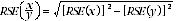10 Considering the example from paragraph 3, of the 300,300 female underemployed part-time workers, 104,200 or 34.7% had insufficient work for 52 weeks and over. The SE of 104,200 may be calculated by interpolation as 3,900. To convert this to an RSE we express the SE as a percentage of the estimate, or 3,900/104,200 = 3.7%. The SE for 300,300 was calculated previously as 6,000, which converted to an RSE is 6,000/300,300 = 2.0%. Applying the above formula, the RSE of the proportion is: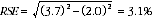11 Therefore, the SE for the proportion of females who have a current period of insufficient work of 52 weeks or more is 1.1 percentage points (=(34.7/100)x3.1). Therefore, there are about two chances in three that the proportion of females who have a current period of insufficient work of 52 weeks or more was between 33.6% and 35.8% and 19 chances in 20 that the proportion is within the range 32.5% to 36.9%.

DIFFERENCES

12 Published estimates may also be used to calculate the difference between two survey estimates (of numbers or percentages). Such an estimate is subject to sampling error. The sampling error of the difference between two estimates depends on their SEs and the relationship (correlation) between them. An approximate SE of the difference between two estimates (x-y) may be calculated by the following formula: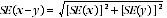13 While this formula will only be exact for differences between separate and uncorrelated characteristics or subpopulations, it is expected to provide a good approximation for all differences likely to be of interest in this publication.

STANDARD ERRORS

 T1 STANDARD ERRORS OF ESTIMATESNSW Vic. Qld. SA WA Tas. NT ACT AustraliaSize of estimate (persons) no. no. no. no. no. no. no. no. no. %100 270 260 190 160 180 100 110 90 100 100.0200 360 340 280 210 240 150 160 140 170 85.0300 430 400 340 250 280 180 210 180 240 80.0500 530 490 440 310 340 220 270 230 340 68.0700 610 550 510 350 390 250 310 260 430 61.41,000 700 640 590 400 450 290 360 290 550 55.01,500 830 740 700 470 520 340 420 310 700 46.72,000 930 830 790 530 580 370 470 330 830 41.52,500 1 000 900 850 550 650 400 500 350 950 38.03,000 1 100 950 900 600 700 400 550 350 1 050 35.03,500 1 150 1 050 1 000 650 700 450 550 400 1 100 31.44,000 1 200 1 100 1 050 700 750 450 600 400 1 200 30.05,000 1 300 1 150 1 100 750 800 500 650 450 1 350 27.07,000 1 500 1 350 1 250 850 950 550 800 500 1 550 22.110,000 1 700 1 500 1 400 950 1 050 650 1 000 600 1 800 18.015,000 2 000 1 750 1 550 1 100 1 200 800 1 350 700 2 100 14.020,000 2 200 1 950 1 700 1 200 1 350 900 1 750 850 2 300 11.530,000 2 550 2 250 1 950 1 400 1 550 1 150 2 400 1 050 2 600 8.740,000 2 850 2 500 2 200 1 600 1 700 1 400 3 050 1 250 2 850 7.150,000 3 100 2 750 2 400 1 800 1 900 1 600 3 650 1 450 3 050 6.1100,000 3 950 3 550 3 250 2 700 2 750 2 250 6 350 1 900 3 850 3.9150,000 4 600 4 350 4 000 3 450 3 650 2 700 . . 2 150 4 500 3.0200,000 5 300 5 050 4 700 4 050 4 400 3 000 . . 2 300 5 050 2.5300,000 6 700 6 500 5 950 5 000 5 500 3 450 . . . . 5 950 2.0500,000 9 350 9 000 8 050 6 250 7 000 . . . . . . 7 500 1.51,000,000 13 900 13 700 11 500 8 000 8 950 . . . . . . 10 550 1.12,000,000 18 750 20 250 15 450 . . 10 350 . . . . . . 15 300 0.85,000,000 23 900 32 400 20 850 . . . . . . . . . . 25 550 0.510,000,000 . . . . . . . . . . . . . . . . 34 100 0.315,000,000 . . . . . . . . . . . . . . . . 38 500 0.3. . not applicable